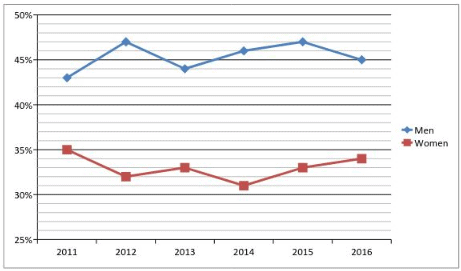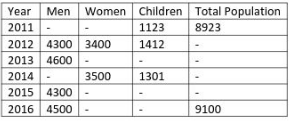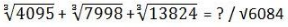# Exam Based Questions - MCQ 3

## 20 Questions MCQ Test Quantitative Aptitude for Competitive Examinations | Exam Based Questions - MCQ 3

Description
This mock test of Exam Based Questions - MCQ 3 for Quant helps you for every Quant entrance exam. This contains 20 Multiple Choice Questions for Quant Exam Based Questions - MCQ 3 (mcq) to study with solutions a complete question bank. The solved questions answers in this Exam Based Questions - MCQ 3 quiz give you a good mix of easy questions and tough questions. Quant students definitely take this Exam Based Questions - MCQ 3 exercise for a better result in the exam. You can find other Exam Based Questions - MCQ 3 extra questions, long questions & short questions for Quant on EduRev as well by searching above.
QUESTION: 1

### Direction (Q. 1-5) Study the following Line Graph and table to answer the following questions. Line Graph percentage of Men and Women having Jobs in that year respectively.Note: Total Population = Men + Women + Children. Q.  If total number of Men and Women who are doing Job in the year 2011 is 3106 then Men population in the year 2011 is?

Solution:

M+W = 8923-1123 = 7800
43M+35W = 3106*100
Then M = 4700

QUESTION: 2

###Note: Total Population = Men + Women + Children. Q.  If the ratio of total number of Job holders in the year 2012 to total number of Job holders in the year 2014 is 1:1, then total population in the year 2014 is?

Solution:

4300*47+3400*32 = X*46+3500*31
X= 4400
Total population = 4400+3500+1301 = 9201

QUESTION: 3

###Note: Total Population = Men + Women + Children. Q.  If the number of Women Job holders in the year 2015 is 1089 and number of Children in 2015 forms 24% of the total population that year then total population in the year 2015 is?

Solution:

Women*33/100 = 1089
Women = 3300
Children/7600+Children * 100 = 24%
C = 2400
Total population = 7600+2400 = 10000

QUESTION: 4Note: Total Population = Men + Women + Children.

Q.

If the total population in the years 2012 is equal to total population in the year 2013,then what is the number of Children in the year 2013 if number of Women job holders are 1089 in the year 2013?

Solution:

Women = 1089*100/33 = 3300
9112 = 4600+3300+Children
Children = 1212

QUESTION: 5Q.

If the ratio of total number of Men and Women in 2016 to the number of Children in 2016 is 11:2 then number of Women having Job in 2016 is?

Solution:

9100 = X+Y
X/Y = 11/2
X = Men + Women = 7700
Women = 3200
Job holders in Women = 32*34 = 1088

QUESTION: 6

What is the Marks secured by Satish in English?

I. Satish wrote exams in Three subjects- Maths, Science and English. He got 72%, 88% in Maths and Science respectively. In total, he secured 84%.

II. Maths exam is out of 50, Science exam is out of 100.

Solution:

84/100 = 36+88+x/150+E

QUESTION: 7

What is the percentage of Illiterates in the Town?

I. A Town having Three Localities X, Y and Z have population in the ratio of 10:11:12
II. In locality X, 70% are literate, in locality Y, 20% are illiterate, in locality Z, 60% are literate

Solution:

10x*30+11x*20+12x*40/33x

QUESTION: 8

2,14,72,252,568,?

Solution:

2*5+2² = 14
14*4+4² = 72
72*3+6² = 252
252*2+8² = 568
568*1+10² = 668

QUESTION: 9

I. 2X – 462X + 528 = 0

II. 2Y – 62(23+3)Y + 432 = 0

Solution:

X = 24/2, 22/2
Y = 24/2, 18

QUESTION: 10

Find the approximate value which should replace the question mark(?)Solution:

(16+20+24)*78 = 4680

QUESTION: 11

5555.1* 11.01 + 444*11.01*11.01 – 6666.1*11.01 – 333*121 + 2222*11.01 = ?

Solution:

121*(505+444-606- 333+202) = 25652

QUESTION: 12

38.85*24.1+(33/16) – (50/32) +0.5 -(2/7) – (2.09*3.34) = ?

Solution:

936+2-1.5+0.5 – 7 = 930

QUESTION: 13

(2196) 1/3 + (344) 1/3 + v80 = (?)² – 500

Solution:

500+29 = 529
? = 23

QUESTION: 14

159.99% of 1798.99 + 14.25% of 281 – 119.99% of 1399.98 = (?)² + 15

Solution:

160% of 1800+ 40- 120% of 1400 -15 = ?²
? = 35

QUESTION: 15

(54656 ÷ 13664) * 589 = (?* 3.001) ÷ 0.5

Solution:

2*588*0.5/3 = ?
? =196

QUESTION: 16

Shopkeeper A bought a 10 chocolate packs having 9 chocolates in each pack at the rate Rs.1.5 per chocolate. Shopkeeper B bought 11 chocolate packs having 8 chocolates in each pack at the rate of Rs. 2 per chocolate. Shopkeeper A made 20% profit by selling all chocolates. Shopkeeper B is chocoholic he ate some chocolates and sold rest of the he chocolates at the same Selling Price as of A. Then how many chocolates did shopkeeper B eat?

Solution:

Shop A
10*9*1.5 = 135
135*120/100 =162
Shop B
11*8*2 = 176
162= 176-2x
x =7

QUESTION: 17

In the year 2015 in VIT University the ratio of ECE, EEE and CSE are in the ratio of 6:7:8. In the year 2016 seats were increased by X%, Y% and Z% respectively in the above branches. By this the number of seats in each branch became equal. Then which of the following combination is true?

Solution:

6*140/100 = 7*120/100 = 8*105/100

QUESTION: 18

A box contains 5 Green 3 Red 4 Black balls. If two balls are drawn at random then what is the probability that there is atleast one Red?

Solution:

(3c 1 * 9c 1 + 3c 2)/12c 2 =5/11

QUESTION: 19

What is the original ratio?

I. 11 is added to numerator and denominator
II. New Ratio of numerator to denominator is 23:33

Solution:

x+11/y+11 = 22/33

QUESTION: 20

What is the total number of Boys and School in the year 2017?

I. In the year 2016 ratio of Boys to Girls is 3:2
II. In the year 2017, they increased by 20% and 15% respectively

Solution:

Based on above two statements total cannot be calculated